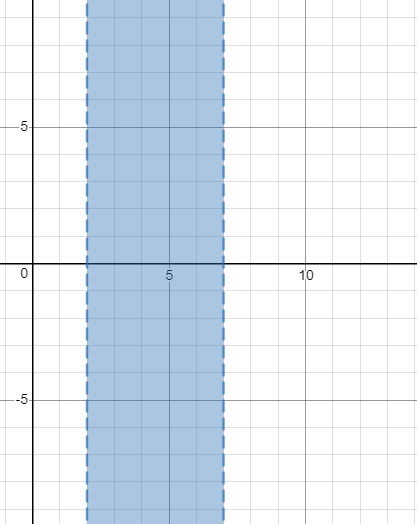# The solution of given linear inequality equation and plot a graph for it.### Precalculus: Mathematics for Calcu...

6th Edition
Stewart + 5 others
Publisher: Cengage Learning
ISBN: 9780840068071### Precalculus: Mathematics for Calcu...

6th Edition
Stewart + 5 others
Publisher: Cengage Learning
ISBN: 9780840068071

#### Solutions

Chapter 1.7, Problem 29E
To determine

## The solution of given linear inequality equation and plot a graph for it.

Expert Solution

2<x<7

### Explanation of Solution

Given:

Linear inequality equation

1<2x5<7

Calculation,

Linear inequality equation,

1<2x5<71+5<2x<74<2x<742<x<72<x<7

Now, graph of 1<2x5<7Conclusion:

Hence, the values of x lies between the 2 and 7.

### Have a homework question?

Subscribe to bartleby learn! Ask subject matter experts 30 homework questions each month. Plus, you’ll have access to millions of step-by-step textbook answers!